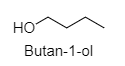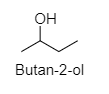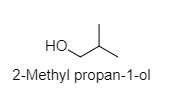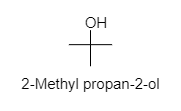QuestionAnswers

# How many alcohol isomers are possible for the formula ${ C }_{ 4 }{ H }_{ 10 }{ O }$?a.) ${ 4 }$b.) ${ 2 }$c.) ${ 3 }$d.) ${ 7 }$Hint: The existence of two or more compounds with the same molecular formula but the different structural formula is known as isomerism; and the compounds themselves are called isomers.

Geometrical isomerism: It is defined as a chemical compound having a similar molecular formula as another yet a different geometric configuration, as when atoms or groups of atoms are attached in various spatial arrangements on either side of a bond or a ring.
There are four geometrical isomers of ${ C }_{ 4 }{ H }_{ 10 }{ O }$ which are given below:

1. Butan-1-ol:2. Butan-2-ol:3. 2-Methyl propan-1-ol:4. Methyl propan-2-ol:As there are four alcohol isomers of ${ C }_{ 4 }{ H }_{ 10 }{ O }$. Hence, the correct option is A.
So, the correct answer is “Option A”.

Characteristics of isomers:
They have the same molecular formula but different structural formulas.
They show similar properties only when they contain the same functional group.
Two isomers can have different boiling points. For example, isomers of pentane branched-chain pentane will have less boiling point than linear because the boiling point depends on the surface area which is more in the case of n-pentane (linear).
Isomers can have different functional groups. For example, aldehyde and ketone are two isomers but they contain various functional groups.

Note: The possibility to make a mistake is that there are seven isomers of ${ C }_{ 4 }{ H }_{ 10 }{ O }$ in which four are alcoholic and 3 are ether isomers. Here, in this question alcohol isomers are asked so in spite of seven, there are four alcohol isomers.
View Notes
Sugar AlcoholIsopropyl AlcoholBenzyl AlcoholGeometric Mean FormulaFormula for AnglesGeometry Formulas For Class 8Geometry Formulas for Class 9Physics Formulas for Class 12 CBSE BoardCBSE Class 12 Physics Atoms FormulaCBSE Class 12 Maths FormulasImportant Questions for CBSE Class 12 Chemistry Chapter 1 - The Solid StateImportant Questions for CBSE Class 12 ChemistryImportant Questions for CBSE Class 12 Chemistry Chapter 5 - Surface ChemistryImportant Questions for CBSE Class 12 Chemistry Chapter 7 - The p-Block ElementsImportant Questions for CBSE Class 12 Chemistry Chapter 8 - The d and f Block ElementsImportant Questions for CBSE Class 12 Chemistry Chapter 16 - Chemistry in Everyday lifeImportant Questions for CBSE Class 12 Chemistry Chapter 3 - ElectrochemistryImportant Questions for CBSE Class 12 Chemistry Chapter 13 - AminesImportant Questions for CBSE Class 12 Chemistry Chapter 14 - BiomoleculesImportant Questions for CBSE Class 12 Chemistry Chapter 2 - SolutionsChemistry Question Paper for CBSE Class 12Chemistry Question Paper for CBSE Class 12 - 2013Chemistry Question Paper for CBSE Class 12 - 2015CBSE Class 12 Chemistry Question Paper 2020Previous Year Question Paper for CBSE Class 12 Chemistry - 2014CBSE Class 12 Chemistry Question Paper 2019 - Free PDFCBSE Class 12 Chemistry Question Paper 2017 - Free PDFCBSE Class 12 Chemistry Question Paper 2018 - Free PDFChemistry Question Paper for CBSE Class 12 - 2016 Set 1 EChemistry Question Paper for CBSE Class 12 - 2016 Set 1 SRD Sharma Solutions for Class 9 Maths Chapter 12 - Heron's FormulaNCERT Solutions for Class 9 Maths Chapter 12 Heron’s FormulaSurface Chemistry NCERT Solutions - Class 12 ChemistryNCERT Solutions for Class 12 Chemistry Chapter 1 The Solid State in HindiNCERT Solutions for Class 12 ChemistryNCERT Solutions for Class 12 ChemistryNCERT Solutions for Class 12 Chemistry Chapter 7 The p-Block Elements In HindiNCERT Solutions for Class 12 Chemistry Chapter 5 Surface Chemistry in HindiNCERT Exemplar for Class 12 Chemistry Chapter-12 (Book Solutions)NCERT Solutions for Class 12 Chemistry Chapter 2